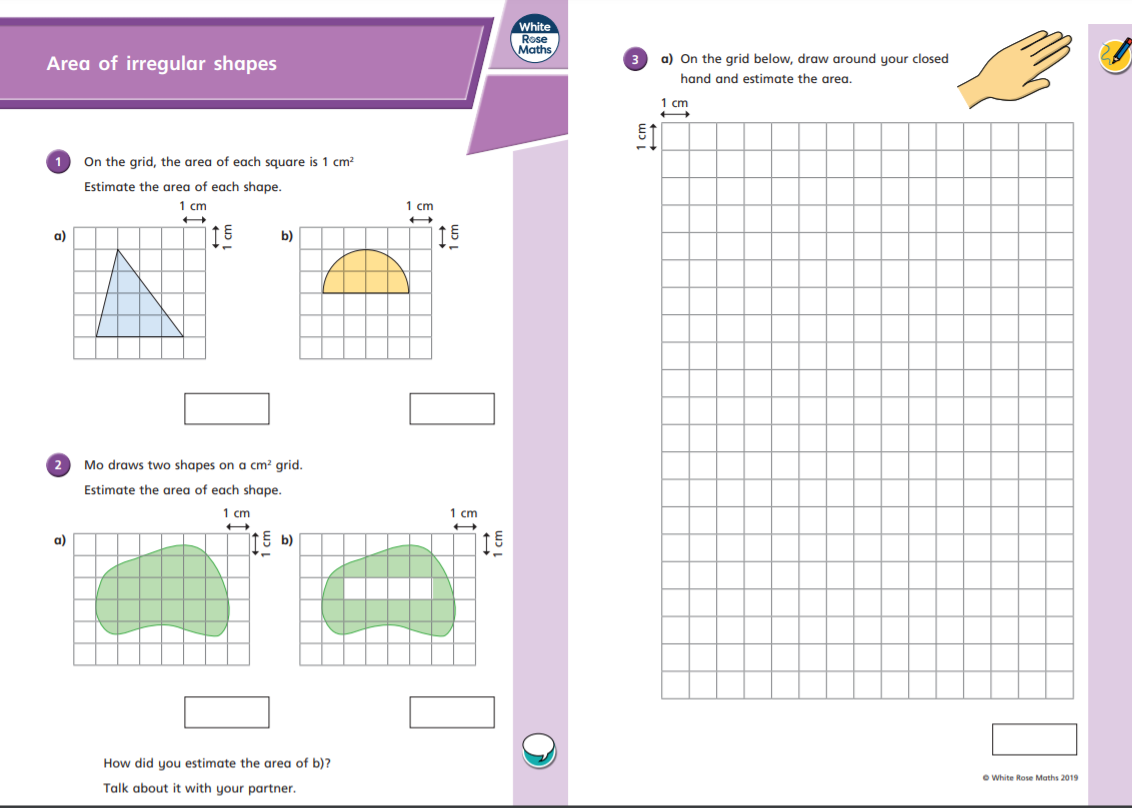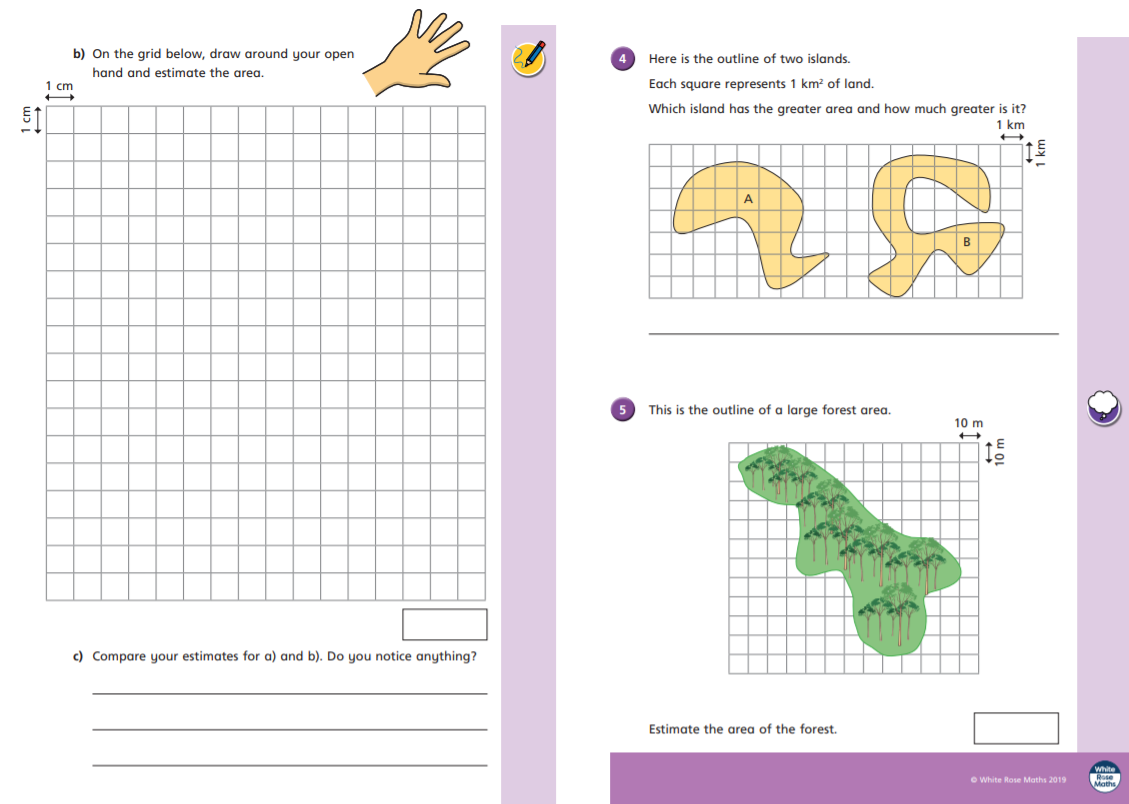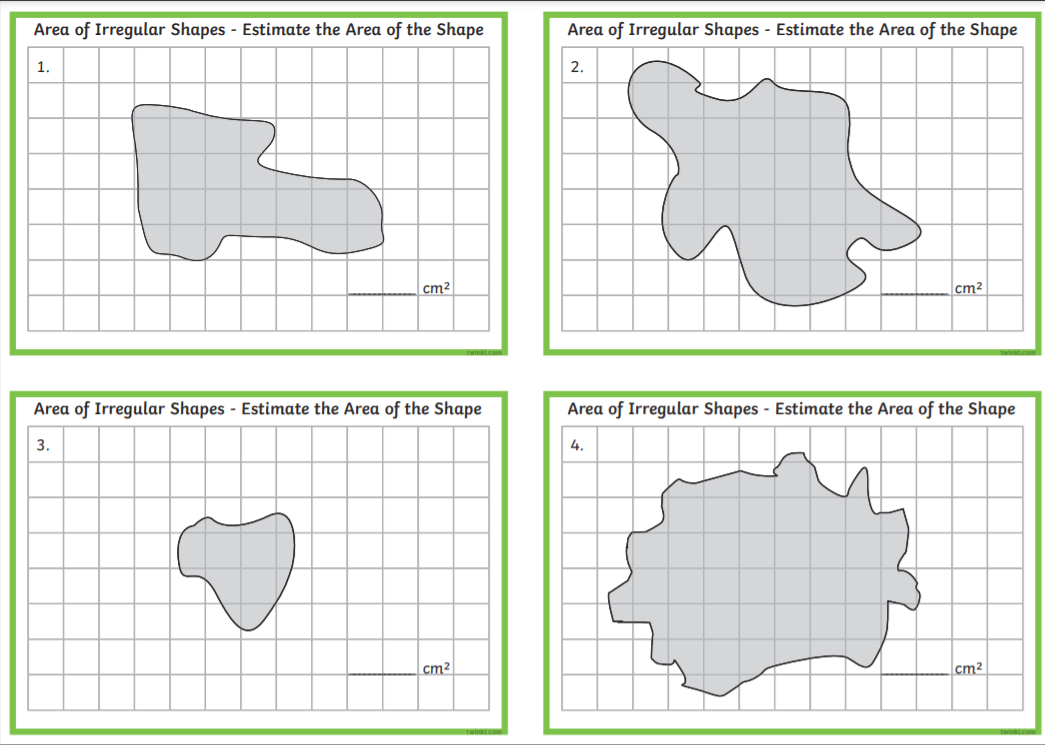# Maths – Thursday 4th March

Today we are learning about the area of irregular shapes. These are shapes where you will have to estimate the area because it cannot be counted or worked out exactly. Watch the video and pause it when required to work on the questions below.CHALLENGE

Estimate the area of these irregular shapes:### 8 thoughts on “Maths – Thursday 4th March”

•4th March 2021 at 9:20 am

Maths:
1. a. 12 squares
b. 8 Squares
2. a. 28 Squares
b. 24 Squares
3. a.
b. I can’t
4. 29km2
35km2
6km2 more
5. I think like about 40-50km2 over

•4th March 2021 at 1:19 pm

Kiran, don’t forget that each square is 100cm squared (not 10).

•4th March 2021 at 12:54 pm

1) a) 8cm2 b) 8cm2
2) a) 20cm2 b) 16cm2 You just do a and then -4cm2
3)
4) A by around 4km2
5) 460m2?

•4th March 2021 at 1:18 pm

For number 5 Franek, don’t forget that each square’s area is 100cm squared (not 10cm squared).

•4th March 2021 at 1:48 pm

Hi, could you explain to me please, what do you mean by 100cm2 when it is 10m2 on the picture for 5?

•4th March 2021 at 7:48 pm

Hello Franek

I actually meant 100m2 not cm!

If you look at the map then it shows that each square is 10 by 10 which means each has an area of 100m2. Therefore you can multiply the amount of squares by 100 to find the area.

Does that make sense now? Sorry to confuse you.

Mr Bushell

•4th March 2021 at 2:02 pm

1.
a) 12 cm squared
b) 8 and a third cm squared

2.
a) 27 and a half cm squared
b) 23 and a half cm squared as you take away 4 cm.

3.
I will send over a picture of A and B

4.
A) 20 km and a half
B) 25 km so Island B is the biggest by 4 and a half km

5.
I would say 5,600 km

•4th March 2021 at 7:53 pm